## 6 Aug 2015

### Priest, Ch2 of Logic: A Very Short Introduction, “Truth Functions–Or not”, summary

[Search Blog Here. Index-tags are found on the bottom of the left column.]

[Central Entry Directory]
[Logic & Semantics, Entry Directory]
[Graham Priest, entry directory]
[Priest’s Logic: A Very Short Introduction, entry directory]

[Bracketed commentary and boldface (unless otherwise indicated) are my own.]

Summary of

Graham Priest

Logic: A Very Short Introduction

Ch.2
Truth Functions – Or Not?

Brief Summary:
Our intuitions about the validity of inferences are often correct, but sometimes they are misleading. One such case is the inference: q, ¬q / p, for example, “The Queen is rich,” “The Queen is not rich,” therefore “Pigs can fly”. Since the conclusion seems logically unrelated, we might erroneously think it is an invalid inference. By rendering these sentences into symbols and computing their truth values, we can see that there is no instance when the premisses are true and the conclusion not-true (false), and thus indeed it is valid. But since there is no situation where both the premisses can be true anyway, it is called vacuously valid. We also learn the truth tables for negation, disjunction, and conjunction, which are based on the truth conditions for these operations. If a sentence is true, then its negation is false, and vice versa. A disjunction is true only if at least one disjunct is true. And a conjunction is true only if both conjuncts are true. But conjunctions and disjunctions in English do not always map perfectly onto these truth tables.

Summary

Previously we discussed the validity of inferences, which comes about when the conclusion follows from the premisses. Now Priest notes that we often sense intuitively when a inference is valid. “There wouldn’t be much disagreement, for example, that the following inference is valid: ‘She’s a woman and a banker; so she’s a banker’” (7).

Yet, our intuitions do not always serve us so well in all cases in logic. In the following image, there are two premisses, found above the line, and the conclusion is below it.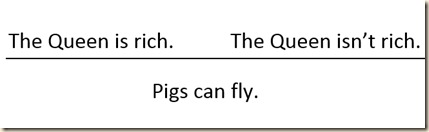Priest writes, “It certainly doesn’t seem valid. The wealth of the Queen – great or not – would seem to have no bearing on the aviatory abilities of pigs” (7).

Now Priest has us consider the following two inferences.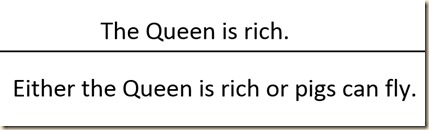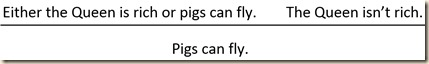He says the first one seems valid. The conclusion contains a disjunction. It has two clauses on either side of the “or”, which are called its disjuncts (8). For a disjunction to be true, one or the other disjunct must be true [and also, both disjuncts may be true]. So, if the premiss, “The Queen is rich” is true, then the conclusion will be true, since that same fact is found in the conclusion, and only it needs to be true. The second example also seems valid. If “The Queen isn’t rich,” as the second premiss states, then in the prior disjunction the other disjunct would be true; thus, “Pigs can fly”. [Note, to be invalid, there would need to be a way for all the premisses to be true and the conclusion false. If the conclusion were false, that would make the second disjunct false, since they are identical. But the second premiss would make the first disjunct false, since they are contraries. That would make the whole first premiss false. The only way to make it true is to affirm that pigs can fly, which would affirm the the conclusion rather than falsify it. Hence it is valid, since there is no way to make the premisses true and the conclusion false.]

So, each of these inferences on their own seems valid. However, when we combine them, they seem to produce an invalid inference.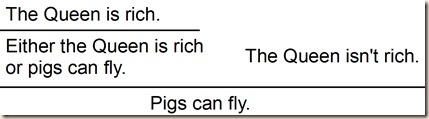[Perhaps the invalidity arises from the second premiss contradicting the first. Since they are contraries, we cannot make all the premises true, since the truth of one means the falsity of the other. But I am not sure what the technical answer is for why this is invalid, and we do not return to this exact inference when later learning how to check them.] But we should be able to chain together inferences that are valid in any situation. We will now examine what has gone wrong.

Priest will begin with an orthodox explanation. We will write “Pigs can fly” as p, and “The Queen is rich” as q. There are two advantages to such a abbreviations: 1) They make the material more compact and easier to analyze, and 2) it really does not matter anyway what content the symbols represent, since we are concerned with their logical relations mostly.

So “Either the Queen is rich or pigs can fly” is now:

Either q or p

Which we can write as:

qp

We will rewrite “The Queen isn’t rich” as “It is not the case that the Queen is rich” by moving the negative particle “not” to the front of the sentence [since we cannot symbolize the negation within the sentence, but we can make a negation operation on the sentence as a whole]. Thus we have, “It is not the case that q,” which can be written as

¬q

which is the negation of q. If we had the conjunction, “The Queen is rich and pigs can fly,” we would write it:

q & p

with q and p being called the conjuncts. “With this machinery under our belt,” we can rewrite the above chain-inference as: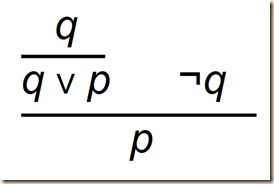Priest will now look at how we may analyze this inference.

He notes first that sentences can be true and they can be false. We use T for truth, and F for falsity. Frege called these “truth values” (9). Now, consider the two sentences a and ¬a. We would normally say that “if one is true, then the other is false, and vice versa. Thus, if ‘The Queen is rich’ is true, ‘The Queen isn’t rich’ is false, and vice versa” (9). We can write that in the following way:

¬a has the value T just if a has the value F.
¬a
has the value F just if a has the value
T.
(9)

These above statements are the truth conditions for negation [since they tell us under what conditions a negated sentence is true or false]. We will assume [for now] that every sentence is either true or false, but not both [a paraconsistent logic, however, would assume that a sentence can be both true and false]. We can depict the truth conditions using a truth table: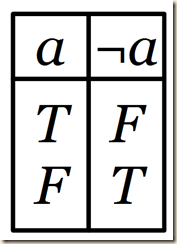Whatever value a is assigned, the corresponding value for ¬a is given at its right.

The disjunction, ∨, is true only if either or both of the disjuncts are true:

ab has the value T just if at least one of a and b has the value T.
a b has the value F just if both of a and b have the value F.
(11)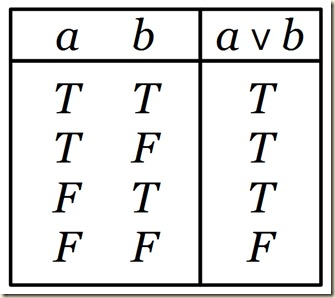For each possible combination of a and b, the truth value of their disjunction is given to the right.

A conjunction is true only if both conjuncts are true, and false otherwise.

a & b has the value T just if both of a and b have the value T.

a & b has the value F just if at least one of a and b has the value F
(11)

[I am not sure why, but the situation where both a and b is false is not mentioned. Perhaps that condition is implied somehow in the others.]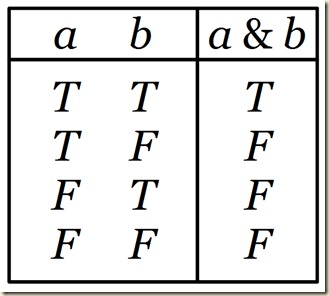In the previous section we wondered what we mean by “situation,” and we return to that question again here.

A natural thought is that whatever a situation is, it determines a truth value for every sentence. So, for example, in one particular situation, it might be true that the Queen is rich and false that pigs can fly. In another it might be false that the Queen is rich, and true that pigs can fly. (Note that these situations may be purely hypothetical!) In other words, a situation determines each relevant sentence to be either T or F. The relevant sentences here do not contain any occurrences of ‘and’, ‘or’ or ‘not’. Given the basic information about a situation, we can use truth tables to work out the truth values of the sentences that do.
(12)

Priest has us consider, then, the situation where p is true, q is false, and r is true. We then wonder, what is the truth value of:

p & (¬rq)

We will perform a sort of mathematical analysis to calculate its truth value on the basis of the values of its parts. r is true, so the ¬r is false. We also know that q is false, so the disjunction is false. p is true, but since the disjunction is false, the entire combination is false. We can determine all such formulas using this step-by-step method.

“Now, recall from the last chapter that an inference is valid provided that there is no situation which makes all the premisses true, and the conclusion untrue (false). That is, it is valid if there is no way of assigning Ts and Fs to the relevant sentences, which results in all the premissses having the value T and the conclusion having the value F” (13). We consider an inference from before,

q / q p

[q therefore q or p].

We can represent the possible value combinations for the premisses and conclusion in the following way: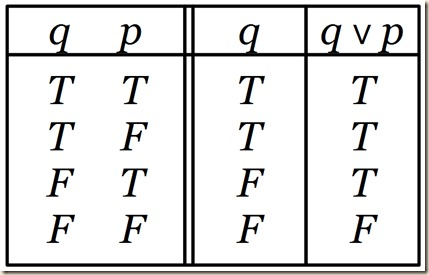The first column just gives all the possible combinations of truth values for q and p. The second column gives the possible values for the premiss, and the third shows the values for the conclusion. We see the inference is valid, since there is no row where the premiss is T and the conclusion F (13).

We now consider the inference:

q p, ¬q / p

which we may depict like this: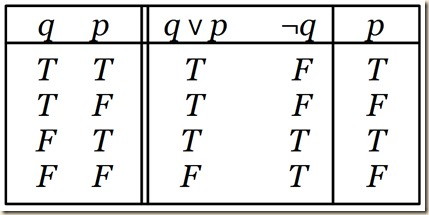We see that this formula is valid.

Now consider the inference we began with:

q, ¬q / p

which we can represent: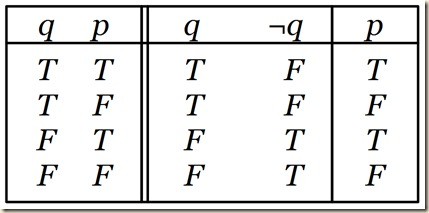Again, the inference is valid; and now we see why. There is no row in which both of the premisses are true and the conclusion is false. Indeed, there is no row in which both of the premisses are true. The conclusion doesn’t really matter at all! Sometimes, logicians describe this situation by saying that the inference is vacuously valid, just because the premisses could never be true together.
(14)

Recall that at the beginning, our intuitions told us this was not a valid inference. But we see now that it is. Thus our intuitions misled us in this case. Perhaps we make this mistake because in real life we never encounter vacuously valid inferences.

Priest will return to this matter in a later chapter. He now examines the “adequacy of the machinery we have used,” since “Things here are not as straightforward as one might have hoped” (15). In our analysis, we determined ¬a on the basis of a, by inverting its truth-value [thus the value of ¬a is a function of a, or we perform a functional operation on a to obtain the value for ¬a]. We similarly found the value of a & b by computing on the basis of the values of a and b. “Logicians call operations that work like this truth functions. But there are good reasons to suppose that ‘or’ and ‘and’, as they occur in English, are not truth functions – at least, not always” (15).

We will consider an example of a tension between the English “and” and the logical operation of conjunction. A conjunction, a & b, is true when both of its conjuncts are true, and it does not matter if it is also formulated b & a. But consider these sentences:

1) John hit his head and fell down.
2) John fell down and hit his head.
(15)

As we can see, there is an order that is implied, so the first can be true while the second one false, and vice versa. Thus in this case we need to know more than just the truth values of the components.

Recall that ‘a or b’ is true if at least one of the disjuncts is true. But consider if your friend tells you:

Either you come now or we will be late

and as a result you decide to come. Now, consider further if you find out that your friend lied to you, and in fact you could have come an hour later. [I am not sure I understand the issue here. Priest’s point will be that “it is not merely the truth values of the disjuncts that are important, but the existence of a connection of a certain kind between them” (16). Perhaps the idea here is that because it is a lie, what makes it false is that the connection between the disjuncts does not hold, regardless of the truth values of the disjuncts themselves. What would make such a formulation true is if the truth of the second disjunct depends on the truth of the first. Thus, the English “or” does not always carry the same logical properties as disjunction in formal logic.]

Priest will leave us to ponder these issues. But, “The material we have been looking at gives us at least a working account of how certain logical machinery functions; and we will draw on this in succeeding chapters, unless the ideas in those chapters explicitly override it – which they will sometimes” (15).

This logical machinery only deals with certain kinds of inferences; however, there are many other kinds of inferences we will look at (15).

Main Ideas of the Chapter

● In a situation, a unique truth value (T or F) is assigned to each relevant sentence.

● ¬a is T just if a is F.

a b is T just if at least one of a and b is T.

a & b is T just if both a and b are T.

(quoted from Priest, 6, boldface his)

From:

Priest, Graham. Logic: A Very Short Introduction. Oxford: Oxford University, 2000.